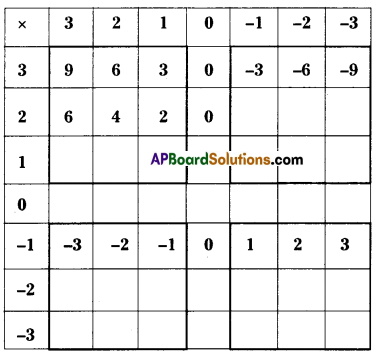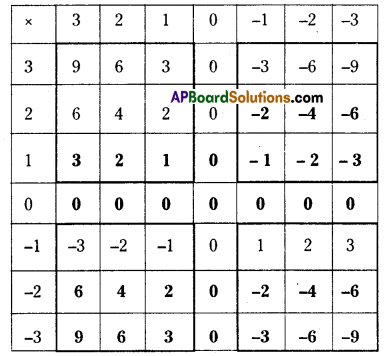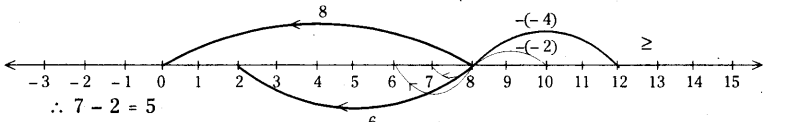# HSSlive: Plus One & Plus Two Notes & Solutions for Kerala State Board

## AP Board Class 7 Maths Chapter 1 Integers InText Questions Textbook Solutions PDF: Download Andhra Pradesh Board STD 7th Maths Chapter 1 Integers InText Questions Book AnswersAP Board Class 7 Maths Chapter 1 Integers InText Questions Textbook Solutions PDF: Download Andhra Pradesh Board STD 7th Maths Chapter 1 Integers InText Questions Book Answers

## Andhra Pradesh State Board Class 7th Maths Chapter 1 Integers InText Questions Books Solutions

 Board AP Board Materials Textbook Solutions/Guide Format DOC/PDF Class 7th Subject Maths Chapters Maths Chapter 1 Integers InText Questions Provider Hsslive

2. Click on the Andhra Pradesh Board Class 7th Maths Chapter 1 Integers InText Questions Answers.
3. Look for your Andhra Pradesh Board STD 7th Maths Chapter 1 Integers InText Questions Textbooks PDF.

## AP Board Class 7th Maths Chapter 1 Integers InText Questions Textbooks Solutions with Answer PDF Download

Find below the list of all AP Board Class 7th Maths Chapter 1 Integers InText Questions Textbook Solutions for PDF’s for you to download and prepare for the upcoming exams:

Try This

Question 1. (Page No. 3)
9 + (-3) =
9 + (- 2) =
9 + C- 1) =
9 + 0 =
9 + 1 =

9 + 2 =
9 + 3 =
9 + 4 =
9 + 5 =
9 + 6 = 15
9 + 7 = 16
Solution:
9 + (- 3) = 6
9 + (- 2) = 7
9 + (- 1) = 8
9 + 0 =9
9 + 1 =10

9 + 2 = 11
9 + 3= 12
9 + 4 = 13
9 + 5 = 14
9 + 6 = 15
9 + 7 = 16

i) When you added a positive integer, in which direction did you move on the number line ? (Page No. 4)
Solution:
When you add a positive integer you move right side on the number line.

ii) When you added a negative integer in which direction did you move on the number line ? (Page No. 4)
Solution:
When you add a negative integer you move left side on the number line.

Question 2.
Sangeetha said that each time you add two integers, the value of the sum is greater than the numbers. Is Sangeetha right ? Give reasons for your answer. (Page No. 4)
Solution:
Sangeetha is always not correct. When we add – 8 and 3 the sum is (- 8) + 3 = – 5 which is less than 3. Therefore when we add two positive integers then only the sum is greater than the numbers.

Try This

Complete the pattern. (Page No. 5)

Question 1.
8-6 = 2
8-5 = 3
8-4 =
8-3 =
8-2 =
8-1 =

8 – 0 =
8 – (- 1) =
8 – (- 2) =
8 – (- 3) =
8 – (- 4) =
Solution:
8-6 = 2
8-5 = 3
8-4 = 4
8-3 = 5
8-2 = 6
8-1 = 7

8-0 =8
8 – (- 1) =9
8 – (-2) =10
8 – (- 3) = 11
8 – (-4) =12

i) When you subtract a positive integer in which direction do you move on the number line ? (Page No. 5)
Solution:
When you subtract a positive integer, you move left side on the number line.

ii) When you subtract a negative integer, in which direction do you move on the number line ? (Page No. 5)
Solution:
When you subtract a negative integer, you move right side on the number line.

Question 2.
Richa felt that each time you subtract an integer from another integer, the value of the difference is lesser than the two numbers. Is Richa right ? Give reasons for your answer. (Page No. 5)
Solution:
Richa may not be correct all times. Richa is correct when we subtract a positive integer, but when we subtract a negative integer the difference is greater than the two numbers.

E.g: 8 – (- 3) = 11 11 > 8 and 11 > – 3
5-(-6) = 11 11 > 5 and 11 >-6
7-(-8) = 15 15 > 7 and 15 > – 8

Do this

Question 1.
Solve the following. (Page No. 7)
i) 2 x – 6 =
Solution:
i) 2 x -6 = -12

ii) 5 x – 4 =
Solution:
ii) 5 x – 4 = – 20

iii) 9 x – 4 =
Solution:
iii) 9 x – 4 = – 36

Activity

Question
Fill the grid by multiplying each number in the first column with each number in the first row. (Page No. 9)i) Is the product of two positive integers always a positive integer ?
ii) Is the product of two negative integers always a positive integer ?
iii) Is the product of a negative and positive integer always a negative integer ?
Solution:i) Yes
ii) Yes
iii) Yes

Try This

(Page No. 10)

a) (- 1) x (- 1) =
b) (- 1) x (- 1) x (- 1) =
c) (- 1) x (- 1) x (- 1) x (- 1) =
d) (- 1) x (- 1) x (- 1) x (- 1) x (- 1) =
Solution
a) (- 1) x (- 1) = 1
b) (- 1) x (- 1) x (- 1) = – 1
c) (- 1) x (- 1) x (- 1) x (- 1) = 1
d) (- 1) x (- 1) x (- 1) x (- 1) x (- 1) = – 1

Do This

Solve the following. (Page No. 12)
i) (- 100) ÷ 5 =
Solution:
-20

ii) (-81)-9 =
Solution:
-9

iii)(- 75) – 5 =
Solution:
– 15

iv) (- 32) – 2 =
Solution:
– 16

v) 125-(-25) =
Solution:
– 5

vi) 80-(-5) =
Solution:
– 16

vii) 64 ÷ (-16)
Solution:
-4

Do This

Question 2.
Compute the following. (Page No. 13)

i) -36 ÷ (-4) =
Solution:
9

ii) (-201) ÷ (-3) =
Solution:
67

iii) (-325) ÷ (- 13)=
Solution:
25

Try This

Question 1.
Can we say that (- 48) + 8 = 48 + (- 8) ? (Page No. 12)
Solution:
Yes.

Check whether
i) 90 ÷ (- 45) and (- 90) ÷ (45)
Solution:
90 ÷ – 45 = – 2 and – 90 45 = – 2
∴ 90 ÷ (-45) = -90 ÷ 45 .

ii) (- 136) ÷ 4 and 136 ÷ (- 4)
Solution:
(- 136) ÷ 4 = – 34 and 136 ÷ (- 4) = – 34
∴ (- 136) ÷ 4 = (136) ÷ (-4)

Question 2.
i) (2 + 5) + 4 = 2 + (5 + 4)
ii) (2 + 0) + 4 = 2 + (0 + 4) (Page No. 15)
Does the associative property hold for whole numbers ? Take two more examples and write your answer.
Solution:
Yes. The associative property holds for whole numbers.
Ex : i) 5 + (6 + 8) = (5 + 6) + 8
ii) 3 + (5 + 4) = (3 + 5) + 4

Question 3.
Add the following. (Page No. 15)
i) 2 + 0
ii) 0 + 3 =
iii) 5 + 0 =
Solution:
i) 2
ii) 3
iii) 5

Question 4.
Similarly, add zero to as many whole numbers as possible. Is zero the additive identity for whole numbers ? (Page No. 15)
Solution:
4+0=4
8+0=8
0+7=7
1+0=1
Therefore zero is the additive identity for whole numbers.

Question 5.
Multiply the following. (Page No. 15)
i) 2 x 3 = …………..
ii) 5 x 4 = …………..
iii) 3 x 6 = …………..
Solution:
i) 6
ii) 20
iii) 18

Question 6.
Similarly, multiply any two whole numbers of your choice. (Page No. 17)
Is the product of two whole numbers always a whole number ?
Solutlion:
3 x 5 = 15
8 x 2 = 16
4 x 3 = 12
Yes, the product of two whole number is always a whole number.

Question 7.
(5 x 2) x 3 = 5 x (2 x 3) (Page No. 18)
Is the associative property true for whole number. Take many more examples and verify.
i) 4 x (3 x 2) = (4 x 3) x 2
4 x 6 = 12 x 2
24 = 24

ii) (8 x 5) x 2 = 8 x (5 x 2)
40 x 2 = 8 x 10
80 = 80

iii) (3 x 6) x 5 = 3 x (6 x 5)
18 x 5 = 3 x 30
90 = 90

Do This

Question 1.
Compute the following : (Page No. 13)
i) -36 ÷ (-4) = −36−4 = 9
ii)(-201) ÷ (-3) = −201−3=2013 = 67
iii) (-325) ÷ (-13) = −325−13 = 25

Question 2.
Is [(- 5) x 2] x 3 = (- 5) x [(2 x 3)] ? (Page No. 18)
Solution:
LHS : [- 10] x 3 = – 30
RHS : (- 5) x (6) =-30
∴ L.H.S = R.H.S

Question 3.
Is [(- 2) x 6] x 4 = (- 2) x [(6 x 4)] ? (Page No. 18)
Solution:
LHS: [-12] x 4 = -48
RHS : (-2) x (24) =-48.
∴ L.H.S = R.H.S

Try This

Question 1.
Take at least 5 different pairs of Integers and see if subtraction is commutative.

i) Consider 5 and -3 (Page No. 20)
5 – ( – 3) = 5 + 3 = 8
(-3) – 5 = -8
Therefore 5 – (-3) ≠ (-3) – 5

ii) Consider 7 and -5
7-(-5) = 7 + 5 = 12
(-5) – 7 = -12
Therefore 7 – (-5) ≠ (- 5) – 7

iii) Consider 2 and – 6
2 – (-6) = 2 + 6 = 8
(-6)- 2 = -8
Therefore 2 – (-6) ≠ (- 6) – 2

iv) Consider -4 and 5
(- 4) – (5) = -9
5- (-4) = 5 + 4 = 9
∴ (-4) – 5 ≠ 5 – (-4)

v) -3 and 8
(-3)- 8 = – 11
8 – (-3) = 8 + 3 = 11
∴(-3)-8 ≠ 8-(-3)
Hence subtraction ¡s not commutative over integers.

Question 2.
Take at least five pairs of integers and check whether they are closed under division. (Page No. 21)
Statement — Inference
i) 12 +- (- 2) = – 6 — Result is an integer

Try This

Question 1.
Show 8 – 6, 8 – 1, 8 – 0, 8 – ( -2), 8 – ( – 4) on the number line. (Page No. 5)
Solution:∴ 8 – 6 = 2; 8 – 1 = 7; 8 – 0 = 8; 8 – (-2) = 10; 8 – (-4) = 12

## Andhra Pradesh Board Class 7th Maths Chapter 1 Integers InText Questions Textbooks for Exam Preparations

Andhra Pradesh Board Class 7th Maths Chapter 1 Integers InText Questions Textbook Solutions can be of great help in your Andhra Pradesh Board Class 7th Maths Chapter 1 Integers InText Questions exam preparation. The AP Board STD 7th Maths Chapter 1 Integers InText Questions Textbooks study material, used with the English medium textbooks, can help you complete the entire Class 7th Maths Chapter 1 Integers InText Questions Books State Board syllabus with maximum efficiency.

## FAQs Regarding Andhra Pradesh Board Class 7th Maths Chapter 1 Integers InText Questions Textbook Solutions

#### Can we get a Andhra Pradesh State Board Book PDF for all Classes?

Yes you can get Andhra Pradesh Board Text Book PDF for all classes using the links provided in the above article.

## Important Terms

Andhra Pradesh Board Class 7th Maths Chapter 1 Integers InText Questions, AP Board Class 7th Maths Chapter 1 Integers InText Questions Textbooks, Andhra Pradesh State Board Class 7th Maths Chapter 1 Integers InText Questions, Andhra Pradesh State Board Class 7th Maths Chapter 1 Integers InText Questions Textbook solutions, AP Board Class 7th Maths Chapter 1 Integers InText Questions Textbooks Solutions, Andhra Pradesh Board STD 7th Maths Chapter 1 Integers InText Questions, AP Board STD 7th Maths Chapter 1 Integers InText Questions Textbooks, Andhra Pradesh State Board STD 7th Maths Chapter 1 Integers InText Questions, Andhra Pradesh State Board STD 7th Maths Chapter 1 Integers InText Questions Textbook solutions, AP Board STD 7th Maths Chapter 1 Integers InText Questions Textbooks Solutions,
Share: